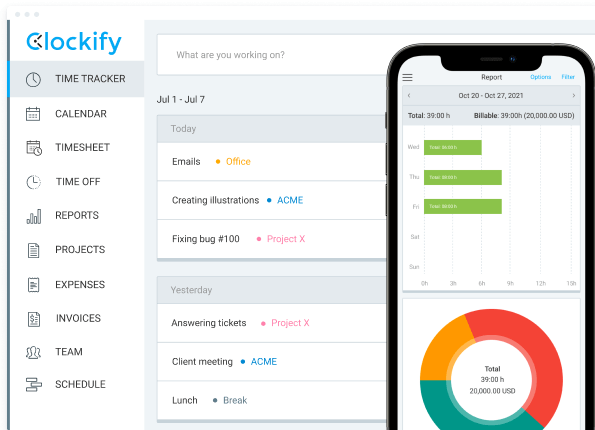# How to calculate and rate employee performance

Ivana Fisic

Last updated on: May 22, 2023

An employee performance rating calculator makes the process of evaluating workers more convenient. Depending on the company’s policy, employers or managers are those who conduct such employee appraisals.

In this blog post, we’ll talk about the benefits of using an employee performance rating calculator, its main components, as well as several calculation methods you can use. You’ll also be able to download and use our free performance rating calculators that accompany each calculation method discussed.

## What is an employee performance rating calculator?

This calculator can include diverse sections of evaluations, like Competencies, Performance goals, Development goals, and others. Inside each of these sections, there are several content items. These items can be individual qualities, skills, or qualifications of employees. You can include any quality or skill you want.

As a manager, you need to examine each content item and each section in order to analyze employee performance and their quality of work. The results of these appraisals are gathered in the performance document. This is an online document that contains employee evaluations for a given period.## The benefits of using an employee performance rating calculator

An employee performance rating calculator makes the process of evaluating workers more convenient. Here are the main reasons why you should try this calculator:

• It’s easy to use and all calculations are done automatically.
• Since you’ll analyze all your employees with the same pattern, you’ll promote transparency and equality among employees.
• The results you get after the appraisals will make it clear whether some employees need improvement in particular areas. But, it will present the top performers as well.

If you’re still not sure what method to use, these are our suggestions:

• An average method without weighting – this is an ideal solution if you need an effortless way to review employee performance, without too many in-depth analyses.
• An average method with weighting – if you want to underline the importance of specific elements of employee performance, choose this method.

## What are the main components of an employee performance rating calculator?

Before we show you how to measure employee output using the calculator, let’s review the basic components of this calculator.

Content item – as we previously mentioned, this is a quality, a skill, or a qualification of an employee. These are qualities that are evaluated.

Sections – each section includes several content items that belong to the same group. For example, the Competency or the Performance goals section.

Rating models – the scale you use to judge content items of an employee. The range is from 1 to 5. Thus, number 5 is a maximum numeric rating.

Competencies – these are all qualities that employees should have when working in a particular position. For instance, skills required for that position, their knowledge of some software or language, or an attribute.

Performance goals – the purpose of these goals is to determine how well an employee is doing towards predetermined goals.

Weight – not all content items have equal value. So, the best way to separate them is to add different weights to each item. Weights are usually added when there are non-quantified activities in the sections, like effort, or teamwork.

The sum of all weights of content items in the same section has to be 100. Aside from items, sections themselves can also have weights. The sum of all weights of sections within a performance document has to be 100.

Section rating – this is the rating you get for a particular section, once you’ve done with its calculations. If a section rating is a decimal number, you need to map it. Then, you’ll get a numeric rating. Plus, on a micro-level, every item has its own numeric rating – item numeric rating.

Decimal score – this is a score you get when you divide an item numeric rating by a maximum numeric rating.

Weighted score – this is a score you get when you multiply a decimal score by weight.

Many companies utilize employee performance ratings because these ratings provide quantitative estimates. In addition, employee ratings are easy to conduct, especially when having a calculator. Furthermore, since the system of evaluations is the same for all workers, these ratings promote equality in the workplace.

If you’re wondering how to measure employee performance with this calculator, we’ll now explore three different sections:

1. Competencies,
2. Performance goals, and
3. Overall summary rating.

## How to calculate employee ratings without weighting?

Organizations can choose whether to perform employee reviews with or without weighting. If you’re looking for an easy, straightforward approach to do the appraisals, you should consider the method without weighting.

Moreover, some experts believe that calculating employee ratings with weighting is too complex and not so effective. Instead, they propose reducing the number of goals and choosing only crucial behaviors in evaluations.

Here are the major steps you need to take when performing a method without weighting.

### The average method without weighting: Competencies

If you want to evaluate the specific competencies of an employee, you can choose the most significant ones, which will be your content items. You can pick whichever quality you find extremely significant.

For example, if you’d like to analyze the productivity levels of your employees, you should include “productivity” as one of the competencies. After evaluations, if you notice that these levels are below appropriate, you should find ways to increase employee productivity in the workplace.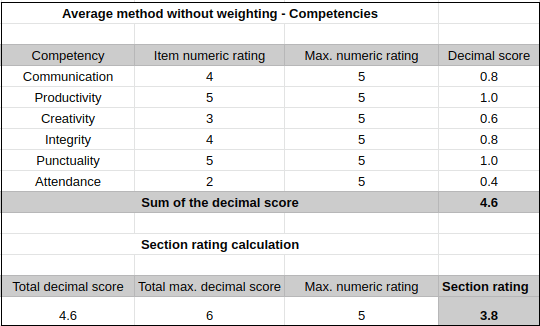As you can see from the table above, we compiled a list of six competencies. The maximum numeric rating for all is 5. Then, we have the item numeric rating that shows how successful an employee is in each area. In this example, we can tell that a worker has high marks for productivity and punctuality, and a very low mark for attendance.

To get decimal scores for these fields, the calculator automatically divides the item numeric rating by the maximum numeric rating:

4 / 5 = 0.8

5 / 5 = 1.0, and so on.

Next, the calculator sums up the total decimal score for all competencies:

0.8 + 1.0 + 0.6 + 0.8 + 1.0 + 0.4 = 4.6

Since you need a rating for the entire section, the calculator takes the total decimal score and divides it by the total maximum decimal score. The total maximum decimal score is equal to the number of skills/competencies that you added to the calculator. In this case, it’s 6.

4.6 / 6 = 0.76

After division, the number gets multiplied by the maximum numeric rating.

0.76 x 5 = 3.8

Finally, the result is the section rating.

Now, if the section rating is a decimal number, you have to map it to get a numeric rating. Here it’s 3.8, so it would be 4. The section rating for this table is 4.

### The average method without weighting: Performance goals

If you wish to examine the performance goals of your employees, you need to select the most significant objectives. These goals should be the ones vital for a specific position and aligned with the company’s goals.

For instance, when analyzing the performance of a sales representative, the crucial goals would be improving customer service, increasing profit, and setting monthly sales goals.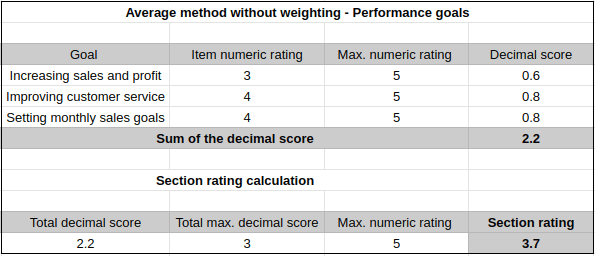The process of calculating the decimal score is the same as in the earlier example.

3 / 5 = 0.6

Once you input all item numeric ratings, the calculator will show you all decimal scores and their total.

0.6 + 0.8 + 0.8 = 2.2

Also, the process of calculating the section rating is identical to the aforementioned. Since there are three goals here, the total maximum decimal score is three.

2.2 / 3 = 0.73

0.73 x 5 = 3.65 ≈ 3.7

In this case, the section rating is 3.7, which means that it will be mapped to 4.

### The overall summary rating without weighting

To be able to see an overall summary rating, the calculations are simple. The calculator determines the average numeric rating for the sections we covered earlier.

The process of calculating an average numeric rating is to get the total of all section ratings. Then, this total is divided by the number of sections in the performance document. So, if there were four sections in the document, the calculator would divide the total number of numeric ratings by four.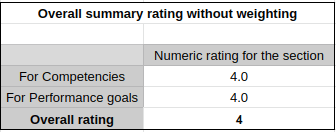In our case, the numeric ratings for both sections are 4 and there are two sections. So, an overall rating is:

(4.0 + 4.0) / 2  = 4

An overall summary rating is 4.

## How to calculate employee ratings with weighting?

Calculating employee ratings with weighting is applied in some situations. For example, when a company believes that specific performance elements are crucial for employees working in the same position. So, the focus of employee appraisals is to evaluate these vital elements. Thus, the sections will get their weights, but so will the content items.

For instance, as an employer, if you want to review the performance of all managers, the elements with higher weight would be leadership and teamwork. On the other hand, if you decide to analyze sales agents, the elements with higher weight would be customer service and communication.

### The average method with weighting: Competencies

When using a method with weighting, the first step is picking the most important competencies an employee should have. The following step is the same as for a method without weighting – adding the maximum numeric rating. Then, you have to think about how an employee is performing compared to the maximum rating. You’ll add these reviews to the item numeric rating column.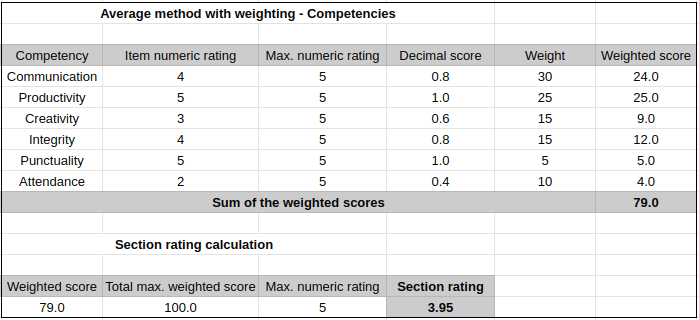The calculator will automatically measure the decimal score, by dividing the item numeric rating by the maximum numeric rating. These decimal scores are identical to the ones from the previous method (calculating competencies without weighting).

As you can see from the table above, competencies have their own weights, depending on their importance. The sum of all these weights is 100.

In our case, the most significant competency here is communication, with a weight of 30. Yet, the least important ones are attendance and punctuality. Speaking of attendance, if your workers are showing low marks in this field, try establishing a rule of employee time tracking.

Next to the weight, there is a weighted score column. To get the weighted score, the calculator multiplies the decimal score by the weight:

0.8 x 30 = 24.0

1.0 x 25 = 25.0, and so on.

Then, the total weighted score is automatically presented below:

24.0 + 25.0 + 9.0 + 12.0 + 5.0 + 4.0 = 79.0

Here’s the process of estimating the section rating – the calculator divides the weighted score by the total maximum weighted score, which is always 100.0.

79.0 / 100 = 0.79

Now, the decimal number should be multiplied by the maximum numeric rating:

0.79 x 5 = 3.95

The section rating is 3.95, which means that it will be mapped to 4.

### The average method with weighting: Performance goals

Employers can choose to emphasize certain performance goals when creating employee appraisals. This means that the Performance goals section will be weighted as well. So, every content item (goal) needs to have its own weight, too.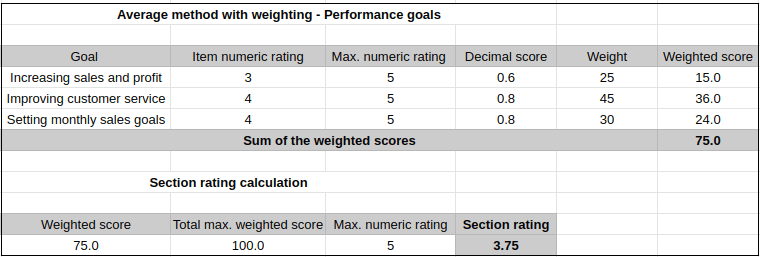Just like we did in a method without weighting, the first step would be to evaluate your employee in every field – for every goal. Once you do that, you’ll have an item numeric rating column ready. Be sure to add the maximum numeric rating, too.

Then, the calculator will divide these ratings by the maximum numeric rating, to get decimal scores:

3 / 5 = 0.6, and so on.

Now that you have decimal scores, you’ll need to determine the weight for every goal in the section. The sum of these weights has to be 100.

Then, the calculator will automatically show you the weighted score, by multiplying the decimal score by its weight:

0.6 x 25 = 15.0, and so on.

Besides, you’ll have a sum of the weighted scores:

15.0 + 36.0 + 24.0 = 75.0

In order to calculate the section rating, the calculator divides the weighted score by the total maximum weighted score:

75.0 / 100 = 0.75

Furthermore, this decimal number gets multiplied by the maximum numeric rating:

0.75 x 5 = 3.75

The section rating is 3.75, so it will be mapped to 4.

### The overall summary rating with weighting

To determine the overall summary rating with weighting, the calculator would need to have decimal scores for all sections. So, you need to input numeric ratings for the weighted sections and maximum numeric rating. The calculator will then present the decimal score for both sections. In our case, numeric ratings are the same, as well as the maximum numeric rating, so the calculation is:

4 / 5 = 0.8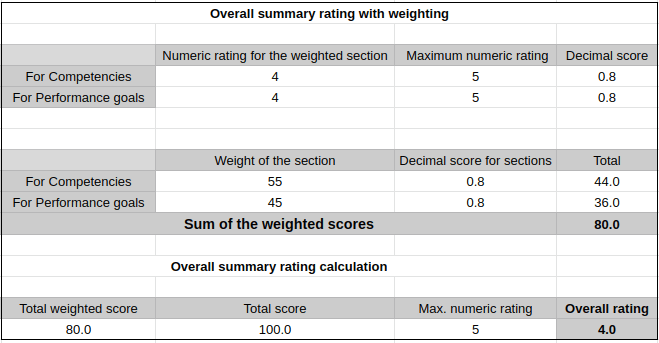We already mentioned that, aside from content items, sections have weights, too. In this case, the Competency has a higher weight (55) than Performance goals (45). So, you need to add their weights to the calculator. That way, the calculator will multiply the weight by the decimal score for the section.

55 x 0.8 = 44.0

45 x 0.8 = 36.0

The sum of the weighted scores is:

44.0 + 36.0 = 80.0

Finally, the calculator uses this sum, divides it by the total score (always 100), and then multiplies by the maximum numeric rating:

80.0 / 100.0 = 0.8

0.8 x 5 = 4

The overall rating is 4.

## Conclusion

An employee performance rating calculator is a handy tool you can use when reviewing employee performance. Once you enter all the necessary data about an employee, such as the most crucial qualities you want to analyze and employee’s marks for these qualities, the calculator will do the rest.

This calculator provides you with two options – either calculating with weighting or without it. You can choose whatever model better suits the needs of your company. So, if you wish to place value on certain competencies, you should use a method with weighting. Otherwise, a model without weighting would be fine.

Finally, seeing the results of these reviews will help you realize if some workers need to improve their competencies. At the same time, the appraisals will reveal the top performers, too.Author: IvanaFisic

Ivana Fisic is a Content Manager at Clockify, overseeing the team of Clockify content writers. She has always been passionate about writing, which is why she has finished her BA in Journalism, at the Faculty of Philosophy, Novi Sad. Ivana is always exploring new methods of how time management can help you organize your workflow more effectively, as well as how you can increase your productivity.

Related posts

## Free time tracker

Time tracking software used by millions. Clockify is a time tracker and timesheet app that lets you track work hours across projects.

FREE FOREVER • UNLIMITED USERS